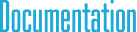#SpatialMatrix6< TYPE > Class Template Reference

## Detailed Description

### template<typename TYPE> class cy::SpatialMatrix6< TYPE >

6D spatial matrix.

This is the general class for 6D spatial matrices. For representing coordinate transformation matrices use SpatialTrans6 instead, since it is more efficient. However, SpatialTrans6 cannot be used for general matrix operations that do not correspond to a coordinate transformation.

`#include <cySpatial.h>`

## Public Member Functions

Constructors
SpatialMatrix6 ()

SpatialMatrix6 (const SpatialMatrix6 &mat)

SpatialMatrix6 (const Matrix3< TYPE > &_R, const Point3< TYPE > &_r)

SpatialMatrix6 (const Matrix3< TYPE > &m11, const Matrix3< TYPE > &m21, const Matrix3< TYPE > &m12, const Matrix3< TYPE > &m22)

SpatialMatrix6 (const SpatialTrans6< TYPE > &tm)

Initialization methods
void Set (const Matrix3< TYPE > &_R, const Point3< TYPE > &_r)

void Set (const Matrix3< TYPE > &m11, const Matrix3< TYPE > &m21, const Matrix3< TYPE > &m12, const Matrix3< TYPE > &m22)

void Set (const SpatialTrans6< TYPE > &tm)

void SetTensorProduct (const SpatialVector6< TYPE > &p1, const SpatialVector6< TYPE > &p2)

void SetIdentity ()

void Zero ()

Unary operators
SpatialMatrix6 operator- () const

SpatialVector6< TYPE > operator* (const SpatialVector6< TYPE > &p) const

SpatialMatrix6 operator* (const SpatialMatrix6 &mat) const

SpatialMatrix6 operator+ (const SpatialMatrix6 &mat) const

SpatialMatrix6 operator- (const SpatialMatrix6 &mat) const

SpatialMatrix6 operator* (TYPE t) const

SpatialMatrix6 operator/ (TYPE t) const

## Public Attributes

Matrix3< TYPE > m 

## Assignment operators

void operator*= (const SpatialMatrix6 &mat)

void operator+= (const SpatialMatrix6 &mat)

void operator-= (const SpatialMatrix6 &mat)

void operator*= (TYPE t)

void SetMatrix (Matrix3< TYPE > &m, const Point3< TYPE > &p1, const Point3< TYPE > &p2)

## § Set() [1/3]

 void Set ( const Matrix3< TYPE > & _R, const Point3< TYPE > & _r )

Sets the matrix as the outer product of two vectors.

## § Set() [2/3]

 void Set ( const Matrix3< TYPE > & m11, const Matrix3< TYPE > & m21, const Matrix3< TYPE > & m12, const Matrix3< TYPE > & m22 )

Sets the matrix as the outer product of two vectors.

## § Set() [3/3]

 void Set ( const SpatialTrans6< TYPE > & tm )

Sets the matrix as the outer product of two vectors.

## § SetTensorProduct()

 void SetTensorProduct ( const SpatialVector6< TYPE > & p1, const SpatialVector6< TYPE > & p2 )

Sets the matrix as the outer product of two vectors.

## § SetIdentity()

 void SetIdentity ( )

Sets the matrix as the outer product of two vectors.

## § Zero()

 void Zero ( )

Sets the matrix as the outer product of two vectors.

## § m

 Matrix3 m

Matrix data in column major order.